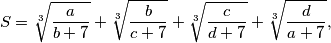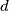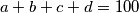### IMO Shortlist 2018 problem A7

Kvaliteta:
Avg: 0,0
Težina:
Avg: 9,0

Find the maximal value ofwhere$a$,$b$,$c$,$d$ are nonnegative real numbers which satisfy$a+b+c+d = 100$.

Izvor: https://www.imo-official.org/problems/IMO2018SL.pdf